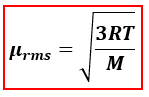# Problem: Which of the following gases will have the largest root mean square speed at 100 °C?1. water2. argon3. oxygen4. methane 5. nitrogen

92% (95 ratings)
###### FREE Expert Solution

We’re being asked to determine the gas that would have the largest root mean square speed at 100°C.

When calculating for root mean square speed (μrms), we will use the following equation:µrms = rms speed,
R = 8.314 J/(mol·K)
T = temperature, K
M = molar mass, kg/mol

Based on the equation for μrms, 3 and R are constants. All of the gases are at the same temperature (100°C). The only difference the gases have is their molar masses (M).

92% (95 ratings)###### Problem Details

Which of the following gases will have the largest root mean square speed at 100 °C?

1. water

2. argon

3. oxygen

4. methane

5. nitrogen

What scientific concept do you need to know in order to solve this problem?

Our tutors have indicated that to solve this problem you will need to apply the Root Mean Square Speed concept. You can view video lessons to learn Root Mean Square Speed. Or if you need more Root Mean Square Speed practice, you can also practice Root Mean Square Speed practice problems.

What is the difficulty of this problem?

Our tutors rated the difficulty ofWhich of the following gases will have the largest root mean...as medium difficulty.

How long does this problem take to solve?

Our expert Chemistry tutor, Dasha took 3 minutes and 27 seconds to solve this problem. You can follow their steps in the video explanation above.

What professor is this problem relevant for?

Based on our data, we think this problem is relevant for Professor Firtha's class at San Bernardino Valley College.